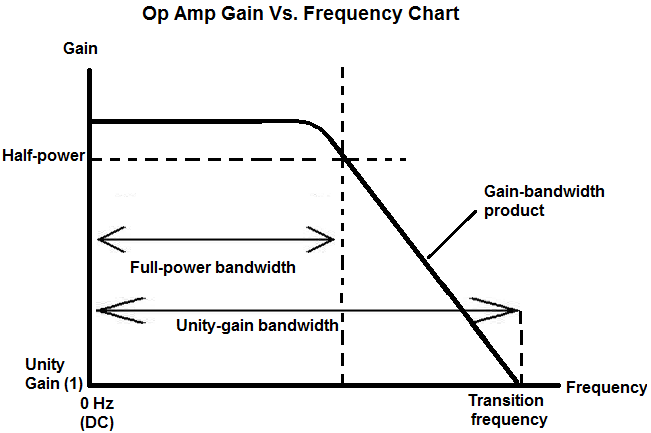﻿ Op Amp- Gain-bandwidth Product# Op Amp Gain-bandwidth ProductThe gain-bandwidth product is gain of an op amp after the half-power point, where the gain of the op amp drops at a constant rate equal to the product of the gain x frequency.

Below is a chart of the gain-bandwidth product of an op amp:The gain-bandwidth product is the region, after the half-power point or full-power bandwidth, where you see a steady, constant decline in the gain of the op amp as the frequency increases.

You can calculate the gain-bandwidth product by the formula:

Gain-bandwidth Product= Gain x Frequency

Beyond the half-power point frequency, the gain falls at a rate such that the product of the gain and the frequency is constant. This constant is the gain-bandwidth product.

An example of gain-bandwidth product calculation: If an op amp has an open-loop gain of 20 at 100KHz, it has a gain of 10 at 200KHz, a gain of 5 at 400KHz, and a gain of 1 at 2MHz. In each calculation, the gain-bandwidth product is equal to the gain x frequency= 2MHz.